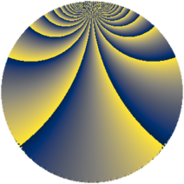# Properties

 Label 648.4.tLevel $648$ Weight $4$ Character orbit 648.t Rep. character $\chi_{648}(37,\cdot)$ Character field $\Q(\zeta_{18})$ Dimension $636$ Sturm bound $432$

# Related objects

## Defining parameters

 Level: $$N$$ $$=$$ $$648 = 2^{3} \cdot 3^{4}$$ Weight: $$k$$ $$=$$ $$4$$ Character orbit: $$[\chi]$$ $$=$$ 648.t (of order $$18$$ and degree $$6$$) Character conductor: $$\operatorname{cond}(\chi)$$ $$=$$ $$216$$ Character field: $$\Q(\zeta_{18})$$ Sturm bound: $$432$$

## Dimensions

The following table gives the dimensions of various subspaces of $$M_{4}(648, [\chi])$$.

Total New Old
Modular forms 1980 660 1320
Cusp forms 1908 636 1272
Eisenstein series 72 24 48

## Trace form

 $$636q + 6q^{2} - 6q^{4} - 12q^{7} + 3q^{8} + O(q^{10})$$ $$636q + 6q^{2} - 6q^{4} - 12q^{7} + 3q^{8} - 3q^{10} - 69q^{14} - 6q^{16} + 6q^{17} + 237q^{20} - 6q^{22} + 12q^{23} - 12q^{25} + 1002q^{26} - 12q^{28} - 12q^{31} - 684q^{32} + 18q^{34} - 840q^{38} - 381q^{40} - 48q^{41} - 885q^{44} - 3q^{46} + 12q^{47} - 12q^{49} - 2817q^{50} + 1371q^{52} - 24q^{55} + 2925q^{56} - 897q^{58} + 630q^{62} - 3q^{64} + 12q^{65} - 2595q^{68} + 999q^{70} - 5958q^{71} - 6q^{73} + 5931q^{74} - 198q^{76} - 12q^{79} - 78q^{80} - 12q^{82} - 8322q^{86} - 1542q^{88} + 6q^{89} - 3243q^{92} - 2031q^{94} + 762q^{95} - 12q^{97} + 972q^{98} + O(q^{100})$$

## Decomposition of $$S_{4}^{\mathrm{new}}(648, [\chi])$$ into newform subspaces

The newforms in this space have not yet been added to the LMFDB.

## Decomposition of $$S_{4}^{\mathrm{old}}(648, [\chi])$$ into lower level spaces

$$S_{4}^{\mathrm{old}}(648, [\chi]) \cong$$ $$S_{4}^{\mathrm{new}}(216, [\chi])$$$$^{\oplus 2}$$